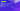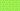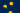Pricing# Understanding the Rule of 70

Written by

When you make an investment, how quickly will it double in size? While growth rates are impossible to predict with 100% accuracy, there are several measurements to help. One handy estimation tool is the rule of 70. We’ll reveal how to calculate doubling time using the rule of 70 below.

## What is the rule of 70?

The rule of 70 offers a way to figure out the doubling time of an investment. In other words, it shows you how many years it will take for your initial deposit to double in size. You’ll need to know the specific rate of return in order to use the rule of 70 or doubling time formula. Although it won’t give you precise results, calculating doubling time is useful for general estimation purposes.

This calculation is also used to compare different investments. If each investment has its own rate of return, you can use the rule of 70 to figure out which one will double your money more quickly.

## When to use the rule of 70

The doubling time rule can be applied to most investments. It’s frequently used when comparing mutual funds or growth rates for investment portfolios. Because it looks at long-term value, it’s often applied to retirement portfolios. Investors want to know that their investment will yield high enough returns within a specific timeframe. By looking at the doubling rate, they can decide whether to diversify their portfolio to increase its growth rate.

The reason why the rule of 70 is popular in finance is because it offers a simple way to manage complicated exponential growth. It breaks down growth formulas into a simple equation using the number 70 alongside the rate of return. This concept is also used to measure the doubling time of a country’s gross domestic product (GDP), which is helpful in comparing growth between different economies.

## How to calculate doubling time?

You can calculate the doubling time of investments using a simple equation. However, to get started you’ll first need to know the investment’s annual rate of growth. You’ll then divide 70 by this growth rate according to the doubling time formula. Here’s what it looks like written out as an equation.

Doubling time formula:

Doubling Time = 70 / Annual Growth Rate

The result shows you the time, in years, it will take for your investment to double. For example, if your mutual fund is growing at an annual rate of 5%, you would divide 70 by 5 to see that it would take approximately 14 years for it to double.

## Rule of 70 vs. rule of 72

The rule of 70 isn’t the only doubling time rule out there. You might also see reference to the rule of 72, as well as the rule of 69. These equations are nearly identical, but they simply use different dividends to come to slightly different results. The rule of 72 leads to longer doubling times, making it a slightly more conservative estimate.

## Rule of 70 and compound interest

One important factor that should be considered when determining an investment’s future growth is compound interest. This is interest calculated over time on an initial or principal amount. Rather than staying static, the interest also includes accumulated interest from previous periods. The higher the number of periods, the higher the value of compound interest. This will impact the time it takes to double your money, so should be looked at alongside the rule of 70.

## The bottom line

Not all investments will double in exactly the amount of time shown through this simple math equation. However, if you want a quick estimate of how long it will take to double your initial investment, the rule of 70 can be of some service. It’s particularly helpful if you need to compare multiple investments, providing useful insight.

#### We can help

GoCardless helps you automate payment collection, cutting down on the amount of admin your team needs to deal with when chasing invoices. Find out how GoCardless can help you with ad hoc payments or recurring payments.

Interested in automating the way you get paid? GoCardless can help

Contact salesSales

Contact sales

+1(415) 523-2279

Support

help@gocardless.com